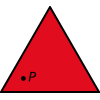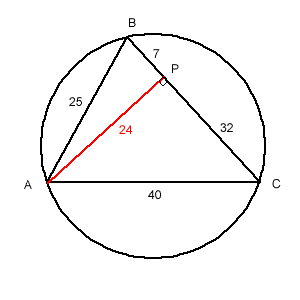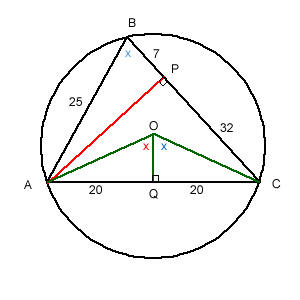#### You may also like### Fitting In

The largest square which fits into a circle is ABCD and EFGH is a square with G and H on the line CD and E and F on the circumference of the circle. Show that AB = 5EF. Similarly the largest equilateral triangle which fits into a circle is LMN and PQR is an equilateral triangle with P and Q on the line LM and R on the circumference of the circle. Show that LM = 3PQ### Triangle Midpoints

You are only given the three midpoints of the sides of a triangle. How can you construct the original triangle?### Three Way Split

Take any point P inside an equilateral triangle. Draw PA, PB and PC from P perpendicular to the sides of the triangle where A, B and C are points on the sides. Prove that PA + PB + PC is a constant.

##### Age 14 to 16 Challenge Level:

Solutions were received from Mary from Birchwood High School; Sana, Jenny, Chris and Rosion of Madras College, St. Andrews; as well as Andrei from School 205 Bucharest.

Some hard work went into these solutions but a little more reflection at the start of the problem could have made it much easier and reduced the consequent errors that appeared.The first thing to look for and notice is Pythagorean triples "hidden" in the sides.

Triangle ABC has AB = 25, AC = 40 and BC=39.

Draw a line from A to BC meeting BC at P so that it divides BC into two parts of lengths BP=7 and PC=32.

AP is perpendicular to BC and AP = 24 (Pythagoras' theorem).

N.B. In triangle APB, $AP^2 = AB^2 - PB^2$

In triangle APC, $AP^2 = AC^2 - PC^2$From the centre O of the circle draw linesOA and OC and the perpendicular from O to AC to meet AC at Q.

Let angle AOQ = x °.

Therefore angle COQ is x (by symmetry)

Angle ABC = 1/2 Angle AOC = x (angle at centre and circumference)

The radius of the circle is OA.

In triangle AOQ
AO = 20/sin x

In triangle ABP
sin x = 24/25

Therefore OA = (20 x 25)/24 = 125/6

Therefore the diameter is 125/3 units (exactly)